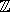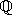## CGAL::Nef_polyhedron_3<Traits>

### Definition

A 3D Nef polyhedron is a subset of the 3-dimensional space that is the result of forming complements and intersections starting from a finite set H of 3-dimensional halfspaces. Nef polyhedra are closed under all binary set operations, i.e., intersection, union, difference, complement, and under the topological operations boundary, closure, and interior.

A 3D Nef polyhedron can be represented by the local pyramids of the minimal elements of its incidence structure. Without going into to much detail, a local pyramid essentially reflects the topologic and geometric situation at a certain location in a point set. For finite polyhedra the minimal elements of the incidence structure are vertices only. This means, that it suffices to model the topological and geometric situation of the vertices. For 3D Nef polyhedra, the local pyramid of a vertex is represented by a planar Nef polyhedra embedded on a sphere.

A Nef_polyhedron_3 consists of vertices V, a sphere map for each vertex in V, edges E, facets F, volumes C, a mark for every item, and an incidence relation on them. Each edge and each facet is represented by two halfedges or two halffacets, respectively.

#include <CGAL/Nef_polyhedron_3.h>

### Parameters

 template < class Nef_polyhedronTraits_3, class Nef_polyhedronItems_3 = CGAL::SNC_items, class Nef_polyhedronMarks = bool > class Nef_polyhedron_3;

The first parameter requires one of the following exact kernels: Homogeneous, Simple_homogeneous, Extended_homogeneous_3 parametrized with Gmpz, leda_integer or any other number type modeling, or Cartesian, Simple_cartesian, Extended_cartesian_3 parametrized with Gmpq, leda_rational, Quotient<Gmpz> or any other number type modeling.

The second parameter and the third parameter are for future considerations. Neither Nef_polyhedronItems_3 nor Nef_polyhedronMarks is specifed, yet. Do not use any other than the default types for these two template parameters.

### Types

 Nef_polyhedron_3::Traits traits class selected for Nef_polyhedronTraits_3. Nef_polyhedron_3::Mark All object (vertices, edges, etc.) are attributed by a Mark. Mark equals bool. Nef_polyhedron_3::size_type size type of Nef_polyhedron_3. Nef_polyhedron_3::Vertex_const_handle non-mutable handle to a vertex. Nef_polyhedron_3::Halfedge_const_handle non-mutable handle to a halfedge. Nef_polyhedron_3::Halffacet_const_handle non-mutable handle to a halffacet. Nef_polyhedron_3::Volume_const_handle non-mutable handle to a volume. Nef_polyhedron_3::SVertex_const_handle non-mutable handle to a svertex. Nef_polyhedron_3::SHalfedge_const_handle non-mutable handle to a shalfedge. Nef_polyhedron_3::SHalfloop_const_handle non-mutable handle to a shalfloop. Nef_polyhedron_3::SFace_const_handle non-mutable handle to a sface. Nef_polyhedron_3::Vertex_const_iterator non-mutable iterator over all vertices. Nef_polyhedron_3::Halfedge_const_iterator non-mutable iterator over all halfeges. Nef_polyhedron_3::Halffacet_const_iterator non-mutable iterator over all halffacets. Nef_polyhedron_3::Volume_const_iterator non-mutable iterator over all volumes. Nef_polyhedron_3::SVertex_const_iterator non-mutable iterator over all svertices. Nef_polyhedron_3::SHalfedge_const_iterator non-mutable iterator over all shalfedges. Nef_polyhedron_3::SHalfloop_const_iterator non-mutable iterator over all shalfloops. Nef_polyhedron_3::SFace_const_iterator non-mutable iterator over all sfaces. Nef_polyhedron_3::SHalfedge_around_svertex_const_circulator non-mutable circulator of shalfedges around a svertex (cw). Nef_polyhedron_3::SHalfedge_around_sface_const_circulator non-mutable circulator of shalfedges around a sface (ccw). Nef_polyhedron_3::SHalfedge_aroud_facet_const_circulator non-mutable circulator of shalfedges around a halffacet (ccw). Nef_polyhedron_3::SFace_cycle_const_iterator non-mutable iterator over the cylces of a sface. Nef_polyhedron_3::Halffacet_cycle_const_iterator non-mutable iterator over the cylces of a halffacet. Nef_polyhedron_3::Shell_entry_const_iterator non-mutable iterator providing an entry to each shell. Nef_polyhedron_3::Object_handle a generic handle to an object. The kind of object (vertex, halfedge, halffacet, volume, svertex, shalfedge, shalfloop, sface) can be determined and the object can be assigned to a corresponding constant handle by one of the following functions: bool assign(Vertex_const_handle& h, Object_handle) bool assign(Halfedge_const_handle& h, Object_handle) bool assign(Halffacet_const_handle& h, Object_handle) bool assign(Volume_const_handle& h, Object_handle) bool assign(SVertex_const_handle& h, Object_handle) bool assign(SHalfedge_const_handle& h, Object_handle) bool assign(SHalfloop_const_handle& h, Object_handle) bool assign(SFace_const_handle& h, Object_handle) where each function returns true iff the assignment to h could be accomplished. Nef_polyhedron_3::Point_3 location of vertices. Nef_polyhedron_3::Segment_3 segment represented by a halfedge. Nef_polyhedron_3::Vector_3 direction of a halfedge. Nef_polyhedron_3::Plane_3 plane of a halffacet lies in. Nef_polyhedron_3::Aff_transformation_3 affine transformation. enum Boundary { EXCLUDED , INCLUDED }; construction selection. enum Content { EMPTY , COMPLETE }; construction selection. Nef_polyhedron_3::Nef_polyhedron_S2 a sphere map. Nef_polyhedron_3::Polyhedron a polyhedral surface.

### Creation

 Nef_polyhedron_3 N ( Content space = EMPTY); creates a Nef polyhedron and initializes it to the empty set if plane == EMPTY and to the whole space if space == COMPLETE. Nef_polyhedron_3 N ( Plane_3 p, Boundary b = INCLUDED); creates a Nef polyhedron containing the halfspace left of p including p if b==INCLUDED, excluding p if b==EXCLUDED. Nef_polyhedron_3 N ( Polyhedron& P); creates a Nef polyhedron, which represents the same point set as the polyhedral surface P does.

### Access Member Functions

 bool N.is_simple () returns true, if N is a 2-manifold. bool N.is_valid () checks the integrity of N . Size_type N.number_of_vertices () returns the number of vertices. Size_type N.number_of_halfedges () return the number of halfedges. Size_type N.number_of_edges () returns the number of halfedge pairs. Size_type N.number_of_halffacets () returns the number of halffacets. Size_type N.number_of_facets () returns the number of halffacet pairs. Size_type N.number_of_volumes () returns the number of volumes. Vertex_const_iterator N.vertices_begin () iterator over all vertices. Vertex_const_iterator N.vertices_end () past-the-end iterator. Halfedge_const_iterator N.halfedges_begin () iterator over all halfedges. Halfedge_const_iterator N.halfedges_end () past-the-end iterator. Halffacet_const_iterator N.halffacets_begin () iterator over all halffacets. Halffacet_const_iterator N.halffacets_end () past-the-end iterator. Volume_const_iterator N.volumes_begin () iterator over all volumes. Volume_const_iterator N.volumes_end () past-the-end iterator.

The following macros are provided: CGAL_forall_vertices(v,N), CGAL_forall_halfedges(e,N), CGAL_forall_edges(e,N), CGAL_forall_halffacets(f,N), CGAL_forall_facets(f,N), CGAL_forall_volumes(c,N) where N is a Nef_polyhedron_3.

 Object_handle N.locate ( Point_3 p) returns a generic handle to the object (vertex, edge, facet or volume) which contains the point p in its relative interior. Nef_polyhedron_S2 N.get_sphere_map ( Vertex_const_iterator v) returns the neighborhood of a vertex modeled by a Nef_polyhedron_S2.

### Point Set Predicates

 bool N.is_empty () returns true, if N is the empty point set. bool N.is_space () returns true, if N is the complete 3D space. bool N == N1 returns true, if N and N1 comprise the same point sets. bool N != N1 returns true, if N and N1 comprise different point sets. bool N < N1 returns true, if N is a proper subset of N1. bool N > N1 returns true, if N is a proper superset of N1. bool N <= N1 returns true, if N is a subset of N1. bool N >= N1 returns true, if N is a superset of N1.

### Unary Set Operations

 Nef_polyhedron_3 N.complement () returns the complement of N . Nef_polyhedron_3 N.interior () returns the interior of N . Nef_polyhedron_3 N.boundary () returns the boundary of N . Nef_polyhedron_3 N.closure () returns the closure of N . Nef_polyhedron_3 N.regularization () returns the regularization, i.e. the closure of the interior, of N . Nef_polyhedron_3 ! N returns the complement of N .

### Binary Set Operations

 Nef_polyhedron_3 N.intersection ( N1) return the intersection of N and N1. Nef_polyhedron_3 N.join ( N1) return the union of N and N1. (Note that ''union'' is a C++ keyword and cannot be used for this operation.) Nef_polyhedron_3 N.difference ( N1) return the difference between N and N1. Nef_polyhedron_3 N.symmetric_difference ( N1) return the symmetric difference of N and N1. Nef_polyhedron_3 N * N1 return the intersection of N and N1. Nef_polyhedron_3 N + N1 return the union of N and N1. Nef_polyhedron_3 N - N1 return the difference between N and N1. Nef_polyhedron_3 N ^ N1 return the symmetric difference of N and N1. void N *= N1 intersects N and N1. void N += N1 unites N with N1. void N -= N1 subtracts N1 from N . void N ^= N1 performs a symmetric intersection of N and N1.

### Operations

void N.clear ( Content space = EMPTY)
make N the empty set if space == EMPTY and the complete 3D space if space == COMPLETE.

void N.transform ( Aff_transformation_3 aff)
applies an affine transformation to N .

void N.convert_to_Polyhedron ( Polyhedron& P)
converts N into a Polyhedron.
Precondition: N is simple.

void
 N.visit_shell_objects ( SFace_const_handle f, Visitor& V)
calls the visit function of V for every item which belongs to the same shell as sf.

### Example

This example program creates two Nef polyhedra - one representing the empty point set, one representing the whole 3D space. The complement of the latter one is computeted and compared with the first one.

```// file: examples/Nef_3/simple.C

#include <CGAL/Gmpz.h>
#include <CGAL/Homogeneous.h>
#include <CGAL/Nef_polyhedron_3.h>

typedef CGAL::Homogeneous<CGAL::Gmpz>  Kernel;
typedef CGAL::Nef_polyhedron_3<Kernel> Nef_polyhedron;

int main() {
Nef_polyhedron N0(Nef_polyhedron::EMPTY);
Nef_polyhedron N1(Nef_polyhedron::COMPLETE);

CGAL_assertion (N0 == N1.complement());
return 0;
}
```